U.S. Department of Transportation
1200 New Jersey Avenue, SE
Washington, DC 20590
202-366-4000

Federal Highway Administration Research and Technology
Coordinating, Developing, and Delivering Highway Transportation Innovations

 REPORT This report is an archived publication and may contain dated technical, contact, and link information
 Publication Number:  FHWA-HRT-12-030    Date:  August 2012
 Publication Number: FHWA-HRT-12-030 Date: August 2012

# Estimation of Key PCC, Base, Subbase, and Pavement Engineering Properties From Routine Tests and Physical Characteristics

PDF files can be viewed with the Acrobat® Reader®

# CHAPTER 5. MODEL DEVELOPMENT (9)

### Compressive Strength Model 5: Long-Term Core Strength Model

This model was developed to estimate the long-term strength of cores taken from a pavement. Data from only the GPS sections were utilized, and they included sections greater than 5 years in age. Strength data at multiple ages were available on some of the sections. A preliminary analysis indicated that pavement age was not a significant factor in the model. In other words, for pavements that have been in service for several years, the strength was more a function of its material parameters than age. This suggests that strength gain is relatively minimal after 5 years or is not noticed in a statistical sense. It then becomes reasonable, or perhaps even necessary, from a statistical standpoint to average the strengths for each section.

The model selected for the long-term strength can be expressed as follows: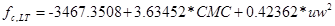#### Figure 161. Equation. Prediction model 5 for fc,LT.

Where:

fc, LT = Long-term compressive strength, psi.

CMC = Cementitious materials content, lb/yd3.

uw = Unit weight, lb/ft3.

The regression statistics for this model are presented in table 30. The model was developed using 201 data points, and the prediction has an R2 value of 18 percent and an RMSE value of 1,179 psi. Table 31 provides details of the range of data used to develop the model. Figure 162 and figure 163 show the predicted versus measured plot and the residual plot, respectively. From observing figure 162, it is evident that this model does not have a good predictive ability, and while there is no significant bias, the error in prediction is fairly high (see figure 163). This model needs to be used with caution, and other means to verify the value would be necessary, such as core tests.

#### Table 30. Regression statistics for long-term core strength model.

 Variable DF Estimate Standard Error t-Value Pr > |t| VIF Intercept 1 -3,467.3508 1,720.49637 -2.02 0.0452 0 Cementitious 1 3.63452 1.38354 2.63 0.0093 1.024 (Unit weight)2 1 0.42362 0.06634 6.39 < 0.0001 1.024

The mode statistics for table 30 are as follows:

• RMSE = 1,179 psi.
• R2 = 0.1803 percent.
• N = 201.

#### Table 31. Range of data used for long-term core strength model.

 Parameter Minimum Maximum Average Cementitious content 354 781 550 Unit weight 134 156 147 Compressive strength 4,315 11,750 7,655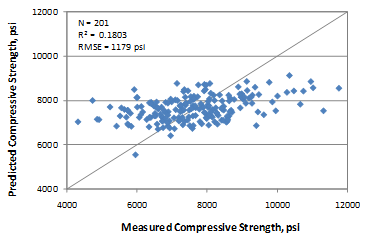#### Figure 162. Graph. Predicted versus measured for long-term core compressive strength model.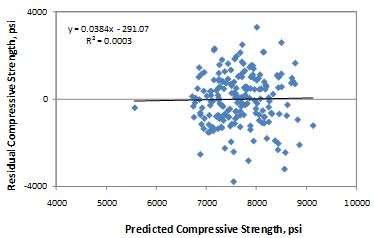### Relative Comparison of All Compressive Strength Models

The compressive strength models presented in this section reproduce the trends present in the datasets used for each correlation. It is highly recommended that a user estimate the strength based on as many models as possible with the information available at the time of analysis. This might provide a fair assessment of the ranges of compressive strength likely for the project and at different ages.

This section presents a comparison of the various models, and the graphs used for this discussion also include raw data plotted with the various relationships. Figure 164 through figure 168 show the relationship between compressive strength and CMC, w/c ratio, and unit weight, respectively. Figure 167 and figure 168 show the strength gain at short- and long-term ages, respectively.

Note that relationships have been plotted for typical values for all variables, and the raw data used in the models do not necessarily lie on the plots.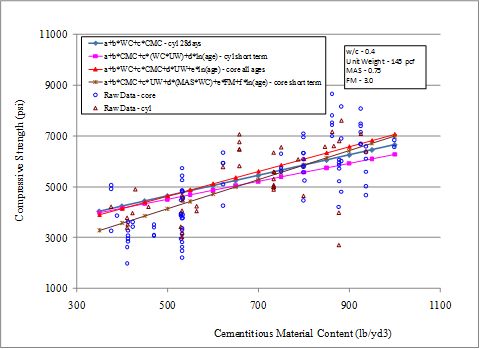#### Figure 164. Graph. Model compressive strength prediction for varying CMC.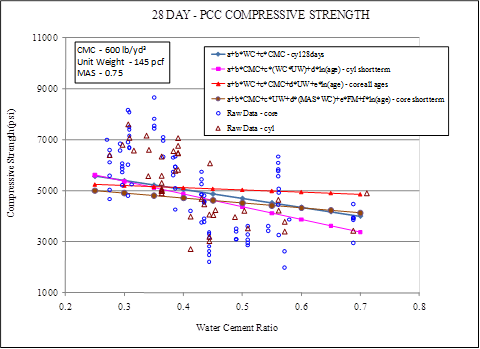#### Figure 165. Graph. Model compressive strength prediction for varying w/c ratio.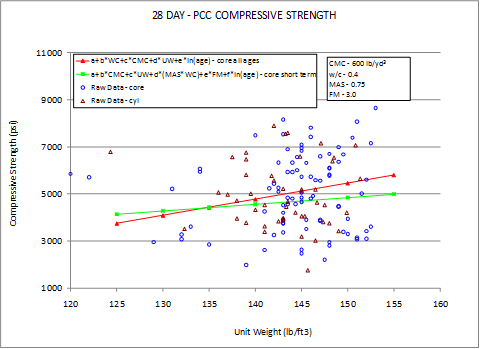#### Figure 166. Graph. Model compressive strength prediction for varying unit weights.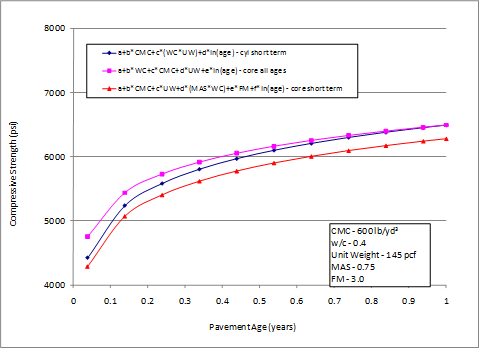#### Figure 167. Graph. Strength gain in the short-term predicted by three models.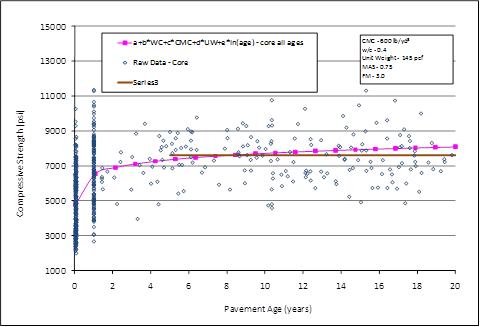#### Figure 168. Graph. Long-term strength gain predicted by the models.

The following observations can be made:

• The predictions are within 700 psi of each other for a given CMC (see figure 164). However, for more typical ranges of cement contents, the predictions are within 300 to 400 psi of each other. The short-term core compressive model has the steepest slope for this relationship.
• Figure 165 suggests that the predictions have a range as high as 1,500 psi for a given w/c ratio, especially at very high w/c ratios. However, within typical ranges (0.3 to 0.5), the models predict within a range of 250 to 800 psi. The range slightly increases at lower w/c ratios. The short-term cylinder strength has the highest slope in this case.
• Based on the trend presented in figure 166, for a given level of unit weight, the compressive strength predictions are within 200 to 300 psi for typical ranges of unit weight (140 to 145 lb/ft3). The prediction can vary by about 800 psi for very high unit weight values. Note that the short-term cylinder compressive strength model has not been included in this plot, as the variable appears as a transformed variable in the model, and its effect cannot be isolated.
• Short-term strength predictions by all models that are relevant to short-term strengths show predictions within 200 to 400 psi of each other. The predictions are closer in value at as the age increases from 14 days to 1 year (see figure 167).
• Figure 168 suggests that the long-term strength predicted by the core all ages model is close to the strength predicted by the long-term model. This is essentially because the data used for strengths at ages greater than 5 years were obtained from the same GPS sections.

These observations illustrate the benefit of comparing predictions made by the various models available to obtain the range of strength that each project or observation could develop. Any other information to substantiate or validate the strength predictions should be utilized whenever possible, such as strength values from other projects that have used similar materials and mix design.

Federal Highway Administration | 1200 New Jersey Avenue, SE | Washington, DC 20590 | 202-366-4000
Turner-Fairbank Highway Research Center | 6300 Georgetown Pike | McLean, VA | 22101